# 2 255 1 is a prime number

What is a prime number?

 A natural number is called a prime number,if it is only divisible by 1 and itself. These are the first 100 prime numbers.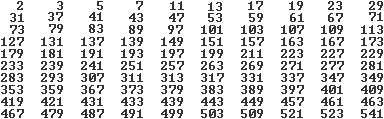Up to 500, 19% of the numbers are prime numbers.

By definition, the number 1 is also a prime number. But it is convenient not to count them.

Christian Gleixner writes to me that one should therefore define: "A number that has exactly two factors is called a prime number."

Natural numbers that are not prime are called composite numbers.

On this page you will find mostly entertaining things on the subject of prime numbers.

Sieve of Eratosthenes Top
There are many ways to find prime numbers.
A computer program found the 100 prime numbers above with the decisive line

If (n / t) = Int (n / t) and i = 2 Then Print n ;.

A method that goes back to Eratosthenes (approx. 276 to approx. 195 BC) is the sieve of Eratosthenes.
 ...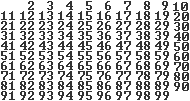... The sequence of the natural numbers is given.Then one crosses off all the numbers that are divisible by 2, 3, 5, ... one after the other.In this case, it's all about the numbers up to 100.It is sufficient to check the prime divisors under sqrt (100) or.

The 25 prime numbers up to 100 remain.

Euclid's reflection on the number of prime numbers  Top
There are an infinite number of prime numbers.
One proof goes back to Euclid and is a famous example of indirect proof.

proof
Assume that the number of prime numbers is finite, namely t.
The t prime numbers should p1, p2, p3, ..., pt with p1= 2, p2= 3, p3= 5, ... be.
Then x = p1* p2* p3* ... * pt+1 a new number which, after construction, is not replaced by any of the prime numbers pi is divisible.
Claim: There is another prime p that divides x, x = a * p. p is necessary of all pj different.

Assume that p = pj (1 <= j <= t). Then pj* b + 1 = a * pj , where b is the product of the remaining t-1 factors.
It also follows from pj* b + 1 = a * pj the equation pj* (b-a) = 1 and further pj =1.
So p is another prime number.

This contradicts the statement that there are only t prime numbers.

Numerical examples
 2*3+1=7 2*3*5+1=31 2*3*5*7+1=211 2*3*5*7*11+1=2311 2*3*5*7*11*13+1=30031=30031=59*509 2*3*5*7*11*13*17+1= 510511=19*97*277 2*3*5*7*11*13*17*19+1=9699691=347*27953

Sequences of prime numbers Top
Derichlet's theorem
Derichlet's theorem below also ensures that there are infinitely many prime numbers.
Every arithmetic sequence an= q * n + r, in which q and r are relatively prime, contains an infinite number of prime numbers.
However, one does not know which members of the sequence are specifically prime numbers.

An example of an arithmetic sequence is an= 4n + 3.
The first 10 prime numbers for an= 4n + 3 :.
 31

There are quadratic terms like an= n² + n + 41, which contain several consecutive prime numbers (after Euler).
The first 10 prime numbers for an= n² + n + 41 are: .....
 nan 041 143 247 353 461 583 697 7113 8131 9151
The sequence of prime numbers can go up to the prime number a40= 1681 to be continued.
But then with a41= 41² + 41 + 41 a composite number.
Oops! Benjamin Rott informs me that a40= 1681 is no longer a prime number. The following applies: 1681 = 41².
The formula also yields consecutive prime numbers for larger values ​​of n.

Further terms of this kind are an = n²-n + 41 and an = n²-79n + 1601.

A series of decimal numbers
The numbers 31, 331, 3331, 33331, 333331, 3333331, 33333331 are prime numbers.
The next number 333333331 is compound. The following applies: 333333331 = 17 * 19607843.

Distribution of the prime numbers Top
Let pi (n) be the number of prime numbers smaller than n, n is a natural number. It applies

 npin code)pi (n) / n 100 000 0005 761 4555,8%
(3) page 81

The table shows: The larger the natural numbers, the greater the number of prime numbers that are counted up to the relevant natural number. The third line is more interesting. The proportion of prime numbers is decreasing more and more. This is also confirmed by further numerical studies.
 ...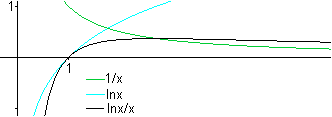..... The graph here only applies to x>>1.......... The famous prime number theorem applies, which approximates the number pi (n) for sufficiently large numbers. We have lim {pi (n) / [ln n / n]} = 1 for n towards infinity. That is, the function f (x) = ln x / x describes the number for sufficiently large natural numbers. - The term ln x increases slowly and steadily, the term 1 / x tends towards 0 for x towards infinity. The composite term ln x / x gets smaller and smaller, but never reaches zero.
For the prime numbers this means that as the numbers get larger they become rarer, but that they never dry up.

The Ulam spiral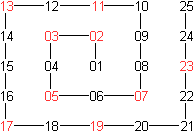...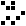... If you arrange the first 25 natural numbers in the form of a spiral and mark the prime numbers in red, you get a figure like the one on the left.In the Ulam spiral, the composite numbers are replaced by white pixels and the prime numbers by black pixels. Surprisingly, the figure shows a structure with increasing numbers. Diagonals are created.

The Ulam spiral can be drawn with an applet by Dario Alpern (URL below). There one reads: The straight line segments are created by the successive prime numbers in quadratic sequences like an= n² + n + 41 (see above). Quadratic terms appear as diagonals in the Ulam spiral.

Goldbach's conjecture Top
In 1742 Christian Goldbach formulated in a letter to Leonard Euler the assumption that every number> 2 can be written as the sum of three prime numbers. Euler replied that he suspected that every even number is a sum of two prime numbers. - At that time the number 1 was counted among the prime numbers.

Today one formulates Euler's conjecture without considering the number 1 as Goldbach's conjecture as follows.
Every even number> 2 can be broken down into two prime numbers in at least one way.

The first decompositions are 4 = 2 + 2, 6 = 3 + 3, 8 = 5 + 3, 10 = 5 + 5 = 3 + 7.

Goldbach's conjecture has not been proven or refuted to this day.

Prime twins Top
These are pairs of prime numbers that differ by the difference of 2.
Up to 100 there are (3, 5), (5, 7), (11, 13), (17, 19), (29, 31), (41, 43), (59, 61) and (71, 73 ).

It is uncertain whether there are infinitely many prime twins.

Special prime numbers Top
Fermat's prime numbers
It's about the numbers an = 2 ^ (2 ^ n) +1. (It means 2 ^ n = 2n.)

The first 7 numbers for an = 2 ^ (2 ^ n) +1
 nan 03 15 217 3257 465537 54294967297 618446744073709551617
The first 5 numbers 3, 5, 17, 257, 65537 are the Fermat prime numbers.
There are no more prime numbers in the sequence. It is also not known to this day whether there are any infinite Fermat prime numbers.
The 6th number 2 ^ (2 ^ 5) + 1 = 4294967297 is a composite number because of 641 * 6700417. This refuted Fermat's (first) conjecture that all numbers with 2 ^ (2 ^ n) +1 are prime.

In the theory of the division of circles, Fermat prime numbers play an important role.

Another odd number: 1031+1 = 11*909090909090909090909090909091

Mersenne prime numbers top
The numbers 2n-1 are called Mersenne numbers.
The first 10 numbers for an= 2n-1:

For certain natural numbers n there are prime numbers.
 89618970019642690137449562111
The question of whether there are infinitely many Mersenne prime numbers has not been decided.

The largest prime numbers are found among the Mersenne numbers.
2 ^ 127-1 was the largest known prime number with digits until 1952.
It has 39 digits and is called 170141183460469231731687303715884105727. Edouard Lucas provided the proof.
(1) page 73f.
After that, with the help of a computer, ever larger Mersenne prime numbers were found.
The last prime number found (2009) is
 # 47 2^42.643.801-1 12,837,064 positions 12.06.2009
You can read more about the search on the websites http://www.mersenne.org/prime.htm and http://www.primzahlen.de.

Composite numbers Top
definition
Numbers that are not prime are called composite numbers.
For them there is always a product representation n = f1* f2 with 1 121 and f2 further decompose and finally arrive at a decomposition into prime factors, n = p1* p2* p3* ... * pt .
The same prime factors can be summarized as powers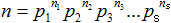.
According to the "main theorem of elementary number theory", every composite number> 1 can be represented as a product of prime numbers, regardless of the sequence.
Numerical example: 300 = 2 * 2 * 3 * 5 * 5 = 2² * 3 * 5²

Almost prime (semiprime, semiprime)
A number that has exactly two prime factors is called an almost prime number.
The first near prime numbers are 4, 6, 9, 10, 14, 15, 21, 22, ...
The smallest almost prime numbers with different prime factors are 6, 10, 14, 15, 21, 22, 26, 33, ...

Sphenic number
A number that has exactly three different prime factors is called a sphenic number.
The sphenic numbers are also near-prime numbers of order 3.
The smallest sphenic numbers are 30, 42, 66, 70, 78, 102, 105, 110, ...

Smooth number
A number that only has prime factors that are less than or equal to k is called a k-even number.
The powers of two are 2-even numbers, 1, 2, 4, 8, 16, 32, 64, 128, ...
The number 2³ * 5³ * 7 = 7000 is 7-smooth.

Numbers games Top
In this chapter results from Lietzmann's book (1) from 1948 are compiled and checked and supplemented with the aid of a computer.
Successive prime numbers

 The sum of the prime numbers from 1 to 59 is 21².The sum of the prime numbers from 3 to 89 is 31².The sum of the prime numbers from 3 to 107 is 37².The sum of the prime numbers from 3 to 131 is 43².The sum of the prime numbers from 5 to 101 is 34².The sum of the prime numbers from 11 to 61 is 22².The sum of the prime numbers from 13 to 37 is 13².The sum of the prime numbers from 37 to 97 is 30².The sum of the prime numbers from 73 to 103 is 25².The sum of the prime numbers from 73 to 109 is 29². 6²=17+1910²=47+5312²=71+7324²=283+29342²=881+883 2³=3+56³=107+10935=41+43+47+53+5927=61+67213=4093+4099 7*11*13*17*19=323 3232*3*5*7*11=11²+13²+17³+19²+23²+29²

Palindromes
 101131151181191 3.13353e+11 7.27758e+11 919929...

Curiosities Top
Mirp numbers (Reversable primes)
A mirp number is a prime number that remains prime if you read it backwards.
An example is 149. The number 941 read backwards is again a prime number.

The quality of being a mere number depends on the job system.
So 149 is not a mirp number in the two-part system: 149 = (10010101)2, but the reverse number is (10101001)2=169.
The strange name comes from prim - mirp. In English, prime becomes emirp.

Mirp numbers below 1000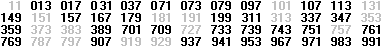The mirp numbers are the black numbers.
The gray numbers are palindromes that could be multiples. It is customary not to count them among them.

Mirp numbers below 100 in the dual system

 (1011)2=11 (1101)2=13 (10111)2=23(11101)2=29 (100101)2=37(101001)2=41 (101011)2=43(101111)2=47 (110101)2=53(111101)2=61(1000011)2=67(1000111)2=71 (1010011)2=83(1100001)2=97..

Truncatable primes
Prime numbers that can be shortened are explained using an example. A prime number that can be trimmed is 739397.
If you remove one digit one after the other from the right, you get the sequence 739397, 73939, 7393, 739, 73, 7.
If you remove one digit one after the other from the left, the result is the sequence 739397, 39397, 9397, 397, 97, 7.
The special thing is that all new numbers are also prime numbers.
There are numbers like 739391133. They can only be right-truncatable.
And there are numbers like 916237547 that can only be left-truncatable.
 7 7 37 3 97 3 9 3 7 3 9 3 9 7 3 9 3 9 7 3 9 3 9 7 9 3 9 7 3 9 7 9 77 7 3 9 3 9 1 1 3 37 3 9 3 9 1 1 37 3 9 3 9 1 17 3 9 3 9 17 3 9 3 97 3 9 37 3 97 37 9 1 6 2 3 7 5 4 7 1 6 2 3 7 5 4 7 6 2 3 7 5 4 7 2 3 7 5 4 7 3 7 5 4 7 7 5 4 7 5 4 74 77

Permutable primes
You swap the digits of one in every way permutable prime number, the new numbers that arise in this way are also prime numbers.
An example is the prime number 733.
The number has the permutations 337 and 373. The two new numbers are also prime numbers.

Cyclic primes
If you swap the digits of one cyclic prime number cyclically, the resulting new numbers are also prime numbers.
An example is the prime number 1193.
The number has the interchanges 1931, 9311 and 3119. The three new numbers are also prime numbers.

Rep-unit primes
The numbers that contain only the digit 1 and are also prime are called Repunit primes.
An example is R2= 11, the next prime number is only R.19=1111111111111111111.

Prime numbers on the internet Top

German

H. B. Meyer
The sieve of Eratosthenes

Wikibooks
Table of prime numbers (2 to 100,000)

Wikipedia
Prime number, sieve of Eratosthenes, prime number twin, Goldbach's conjecture, Dirichletscher prime number theorem, Ulam spiral,
Small Fermatsch theorem, Mirp number, Truncatable Prime, Repunit, Sphenic number, Gaussian number
Also in alphabetical order

English

Dario Alpern
Ulam's Spiral (applet)

Eric W. Weisstein (MathWorld)
Prime Number, Twin Primes, Prime Number Theorem, Goldbach Conjecture, Emirp, Semiprime, Emirpimes,
Truncatable Prime, Smooth Number, Rough Number, Semiprime,
Economical Number, Equidigital Number, Wasteful Number,

GIMPS Home
Great Internet Mersenne Prime Search

Robert Sacks
Number spirals

The On-Line Encyclopedia of Integer Sequences
The prime numbers. A000040
Mersenne primes. A000668
Fermat numbers. A000215
Primes of form n ^ 2 + n + 41. A155884
Repunits: (10 ^ n - 1) / 9. A002275
Emirps. A006567
Primes that are both left-truncatable and right-truncatable. A020994
Absolute primes: every permutation of digits is a prime. A003459
Semiprimes (or biprimes): products of two primes. A001358
Products of 3 distinct primes. A007304
Additive primes: sum of digits is a prime. A046704

Wikipedia
Prime number, Ulam spiral, Twin prime, Goldbach's conjecture, Fermat's little theorem, Dirichlet's theorem on arithmetic progressions, Emirp, Truncatable prime, Permutable prime, Repunit prime, Smooth number, Rough number,
Semiprime, Sphenic number, Gaussian integer
More in alphabetical order

credentials Top
(1) Walter Lietzmann: Oddities in the Realm of Numbers, Bonn 1948
(2) Maximilian Miller: Solved and unsolved mathematical problems, Leipzig 1982
(3) Jan Gullberg: Mathematics- From the Birth of Numbers, New York, London 1997 (ISBN0-393-04002-X)

Feedback: Email address on my main page

URL of my homepage:
http://www.mathematische-basteleien.de/